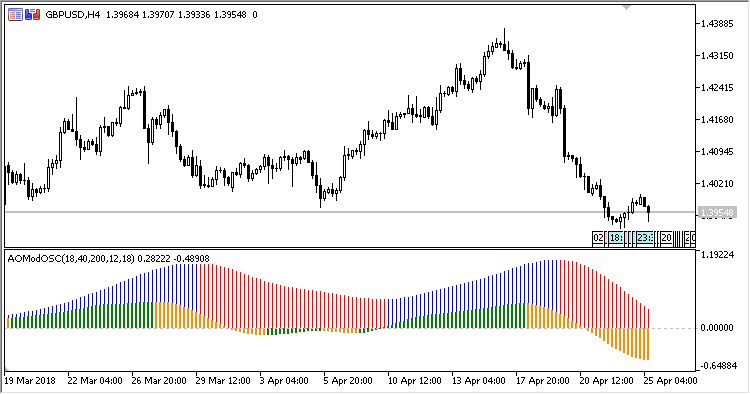# AwesomeMod – indicator for MetaTrader 5

• A+
category：MT5 INDICATORS

The indicator represents two Awesome Oscillators with different smoothing periods, AO Fast and AO Slow, calculated based on three components: Fast EMA, Medium EMA, and Slow EMA.

It has six input parameters:

• Fast EMA period - fast EMA period for calculating the AO;
• Medium EMA period - medium EMA period for calculating the AO;
• Slow EMA period - slow EMA period for calculating the AO;
• AO. Fast MA period - fast AO smoothing period;
• AO. Slow MA period - slow AO smoothing period;
• Applied price - the price of EMA calculating.

Calculations:

```AO Fast = MVA(100*(Fast_EMA/Medium_EMA - 1), AO_Fast_Period)
AO Slow = MVA(100*(Medium_EMA/Slow_EMA - 1), AO_Slow_Period)
```